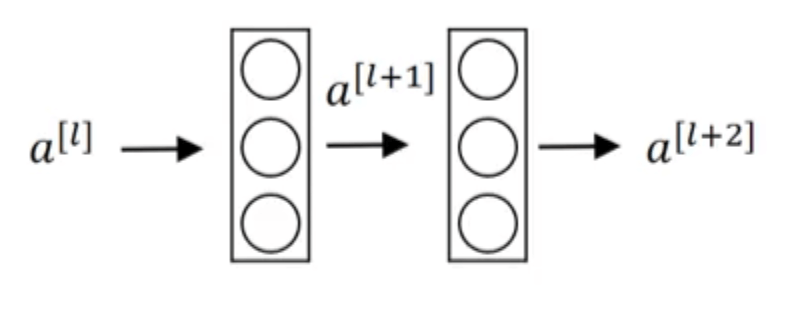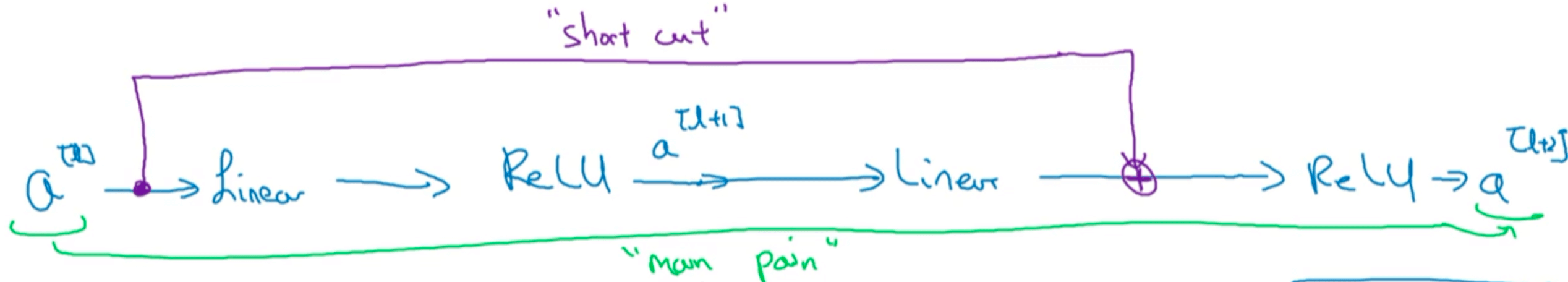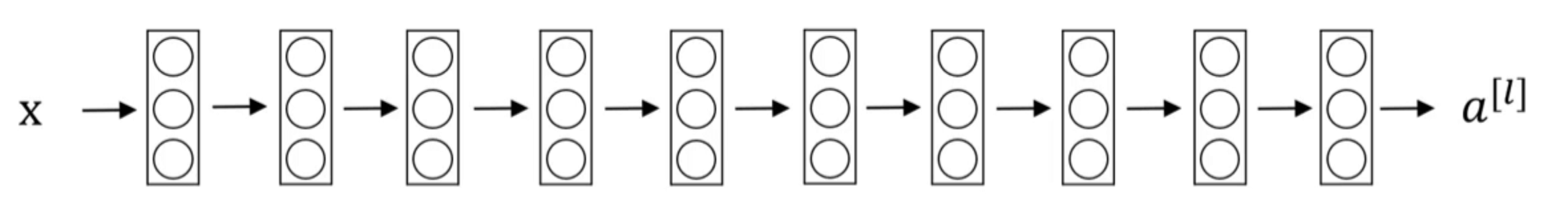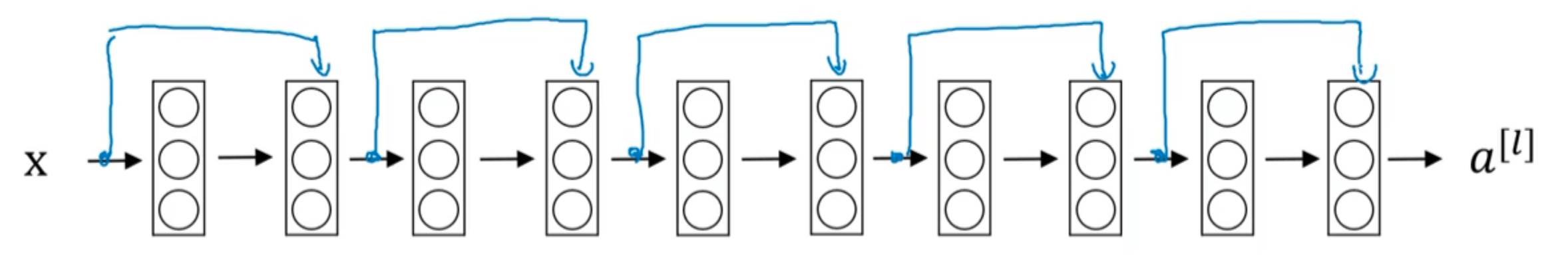# 【深度学习基础】第三十课：残差网络ResNets

## ResNets

Posted by x-jeff on July 25, 2020

【深度学习基础】系列博客为学习Coursera上吴恩达深度学习课程所做的课程笔记。

# 1.前言

1. 计算资源的消耗。
2. 模型容易过拟合。
3. 梯度消失/梯度爆炸问题的产生。

# 2.残差网络的结构1. $z^{[l+1]}=W^{[l+1]}a^{[l]}+b^{[l+1]}$
2. $a^{[l+1]}=g(z^{[l+1]})$
3. $z^{[l+2]}=W^{[l+2]}a^{[l+1]}+b^{[l+2]}$
4. $a^{[l+2]}=g(z^{[l+2]})$1. $z^{[l+1]}=W^{[l+1]}a^{[l]}+b^{[l+1]}$
2. $a^{[l+1]}=g(z^{[l+1]})$
3. $z^{[l+2]}=W^{[l+2]}a^{[l+1]}+b^{[l+2]}$
4. $a^{[l+2]}=g(z^{[l+2]}+a^{[l]})$# 4.参考资料